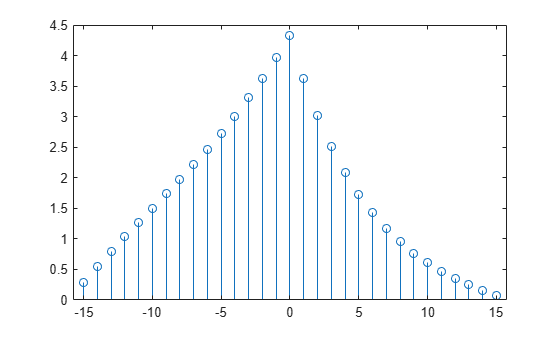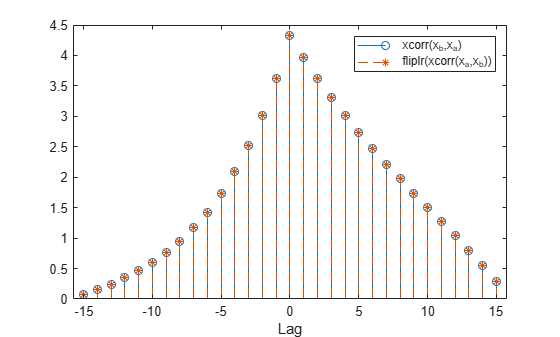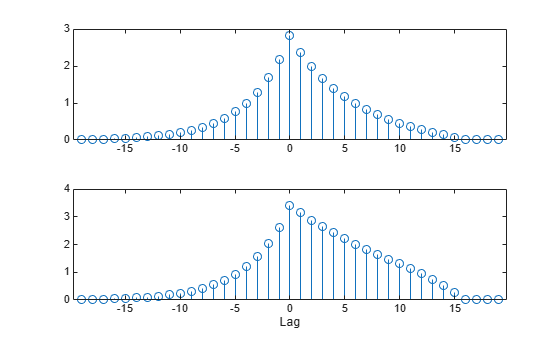# Cross-Correlation of Two Exponential Sequences

Compute and plot the cross-correlation of two 16-sample exponential sequences, ${x}_{a}=0.8{4}^{n}$ and ${x}_{b}=0.9{2}^{n}$, with $n\ge 0$.

```N = 16; n = 0:N-1; a = 0.84; b = 0.92; xa = a.^n; xb = b.^n; r = xcorr(xa,xb); stem(-(N-1):(N-1),r)```Determine $c$ analytically to check the correctness of the result. Use a larger sample rate to simulate a continuous situation. The cross-correlation function of the sequences ${x}_{a}\left(n\right)={a}^{n}$ and ${x}_{b}\left(n\right)={b}^{n}$ for $n\ge 0$, with $0, is

```fs = 10; nn = -(N-1):1/fs:(N-1); cn = (1 - (a*b).^(N-abs(nn)))/(1 - a*b) .* ... (a.^nn.*(nn>0) + (nn==0) + b.^-(nn).*(nn<0));```

Plot the sequences on the same figure.

```hold on plot(nn,cn) xlabel('Lag') legend('xcorr','Analytic')```Verify that switching the order of the operands reverses the sequence.

```figure stem(-(N-1):(N-1),xcorr(xb,xa)) hold on stem(-(N-1):(N-1),fliplr(r),'--*') xlabel('Lag') legend('xcorr(x_b,x_a)','fliplr(xcorr(x_a,x_b))')```Generate the 20-sample exponential sequence ${x}_{c}=0.7{7}^{n}$. Compute and plot its cross-correlations with ${x}_{a}$ and ${x}_{b}$. Output the lags to make the plotting easier. `xcorr` appends zeros at the end of the shorter sequence to match the length of the longer one.

```xc = 0.77.^(0:20-1); [xca,la] = xcorr(xa,xc); [xcb,lb] = xcorr(xb,xc); figure subplot(2,1,1) stem(la,xca) subplot(2,1,2) stem(lb,xcb) xlabel('Lag')```